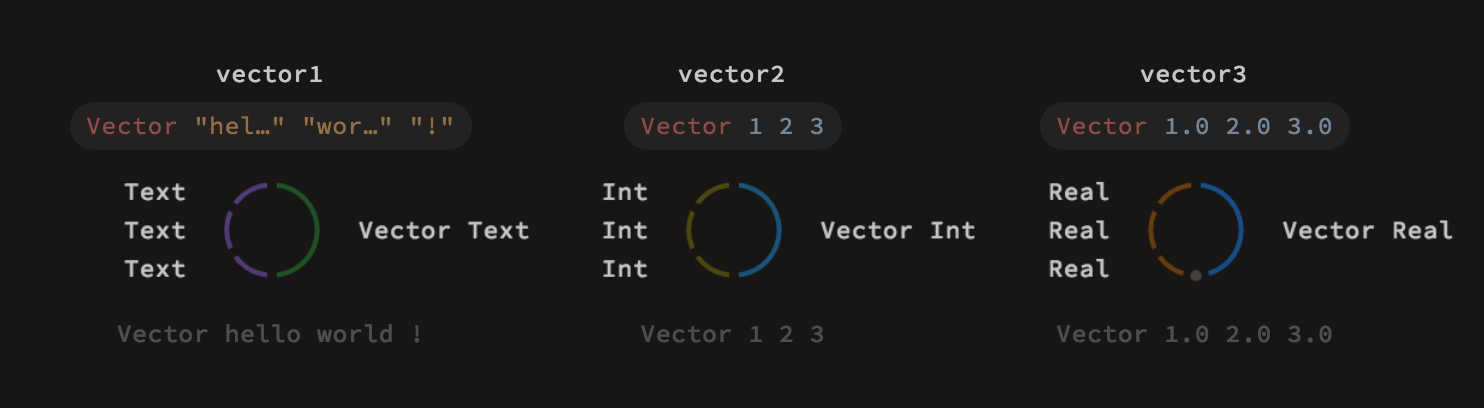# Parametric Polymorphism

## Type parameters

Classes in Luna can also take type parameters, making them polymorphic in some values. For an example, consider the previous definition of `Vector`:

``````class Vector:
x y z :: Real
``````

What if we needed a `Vector` of `Int`s? In the current approach, it would require us to create another class just for this purpose. We can, however, add a type parameter to the `Vector` class:

``````class Vector a:
x y z :: a
``````

Equipped with this definition, we can create vectors containing elements of any type, such as `Real`s, `Int`s, `Bool`s etc.

``````Vector "hello" "world" "!" :: Vector Text
Vector 1 2 3 :: Vector Int
Vector 1.0 2.0 3.0 :: Vector Real
``````## Constrained methods

It is also possible to implement methods that assume some additional properties of the type `a` (such as supporting arithmetic operations, or having defined some other methods). Once you use such properties, Luna typechecker automatically keeps track of them and checks whether they are satisfied. For example:

``````class Vector a:
x y z :: a
def dotProduct that:
self.x * that.x + self.y * that.y + self.z * that.z
``````

The `dotProduct` method will work with any elements supporting addition and multiplication, so using it with `Int`s or `Real`s is fine, while using it with `Text` results in a type error.

``````Vector 1 2 3 . dotProduct (Vector 4 5 6) # returns 32 :: Int
Vector 1.0 2.0 3.0 . dotProduct (Vector 4.0 5.0 6.0) # returns 32.0 :: Real
Vector "hello" "world" "!" . dotProduct (Vector "foo" "bar" "baz") # does not compile
``````